# How to Use the IF Function in Google Sheets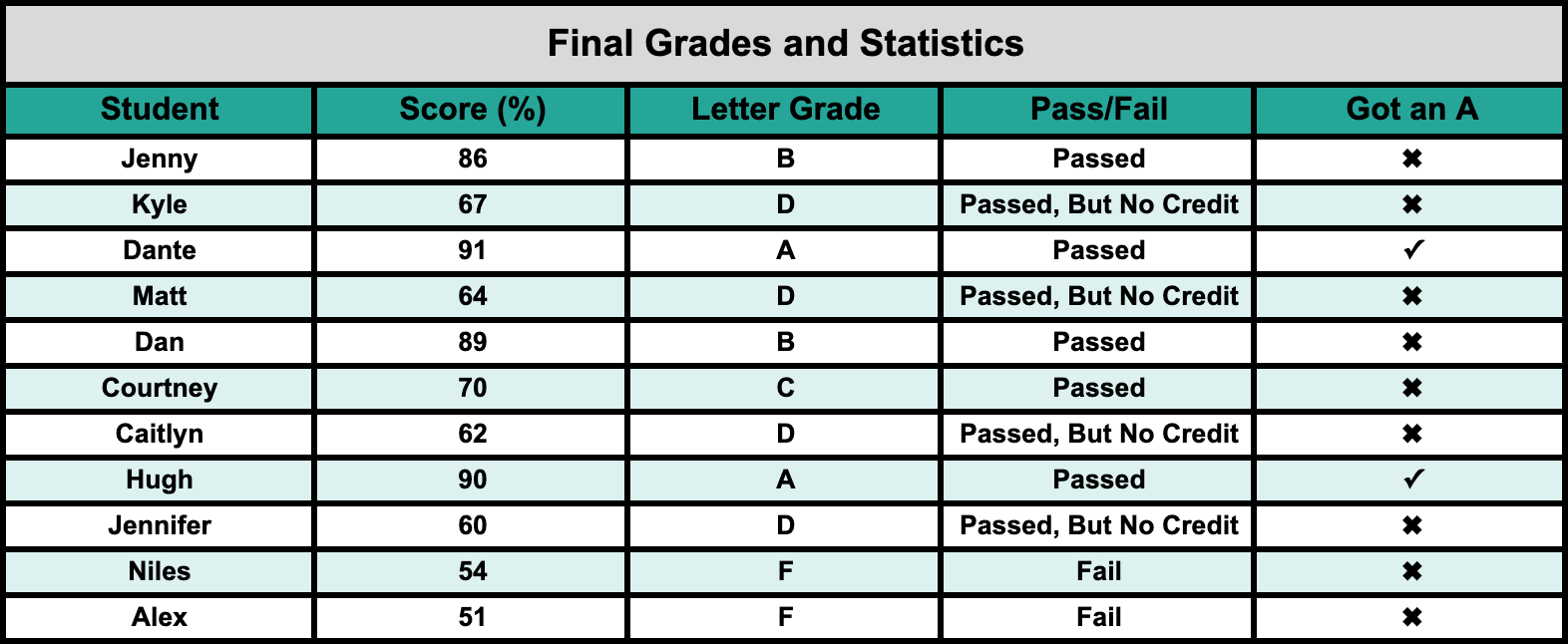The IF function in Google Sheets (somewhat like the IFS function) lets you command your data with more than just a simple True/False statement. If you know how to properly use it, you will give Google Sheets the ability to rationalize data for you, and save yourself countless hours of analysis.

I will show you a basic walkthrough for how it works, followed by a more complex example.

## Basic Walkthrough

First, lets look at an extremely simple example. I have a finance chart for my imaginary pool business where I hire teenagers to manage my stands at the pool.

### Step #1: If-Then-Else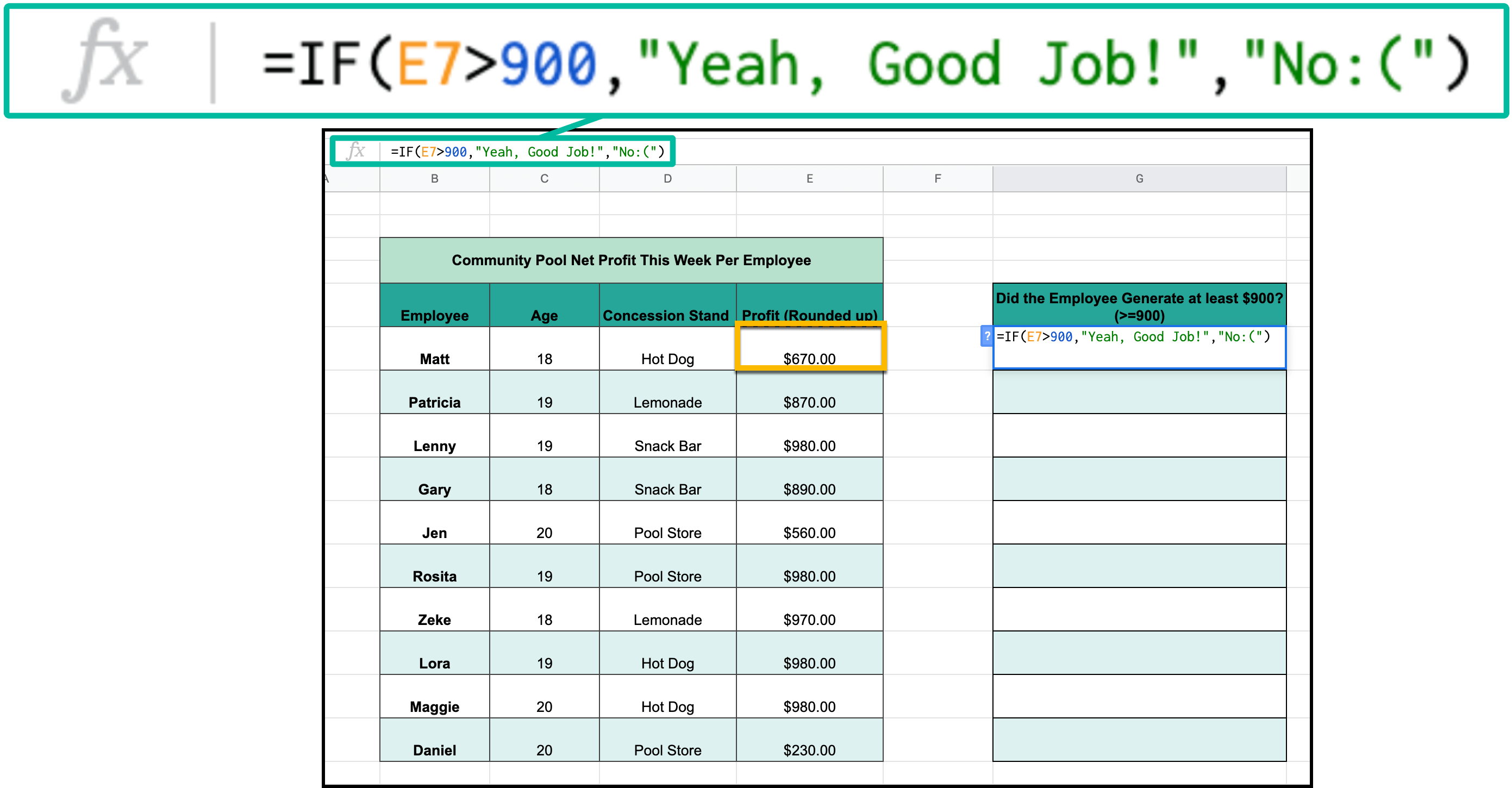I want to quickly sort out what workers have not made me at least \$900 in total profit this week. I will do so with the IF function

the way the equation works is in this order

IF:

=IF(E7>900,": This sets the conditional statement that if the cell has a starting value greater than 900, the first command will take effect.

THEN:

""Yeah, Good Job!",":If the cell is greater than 900, then the message "Yeah, Good Job!" will be displayed.

ELSE:

""NO :(")": or else if the cell condition is not met, then the second command will execute, and a message reading "No :(" will be displayed in the cell instead.

### Step #2: Autofill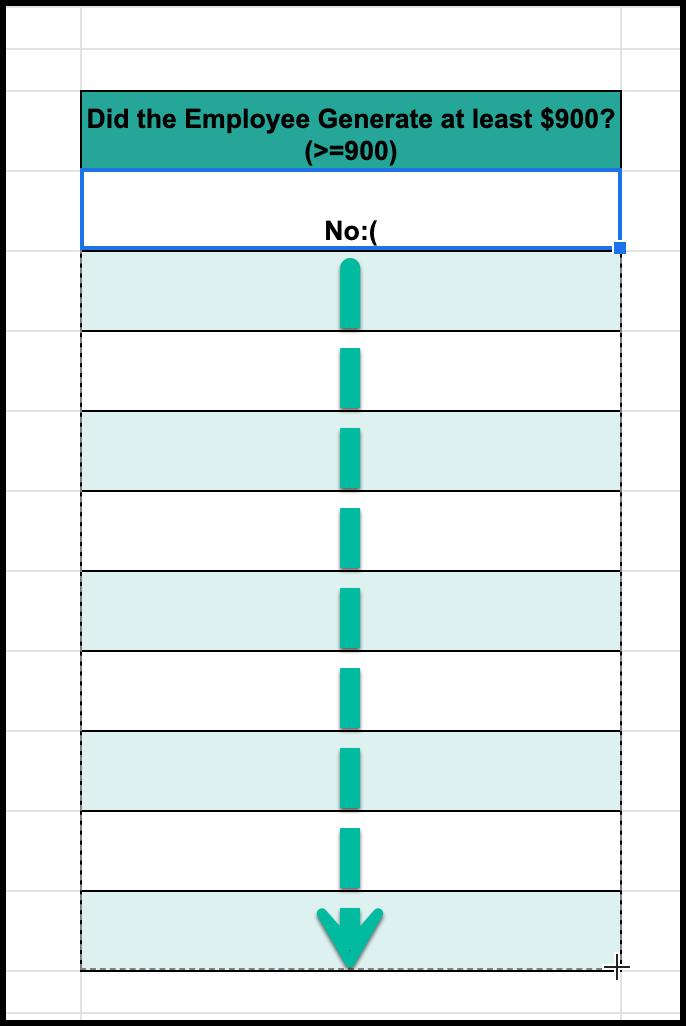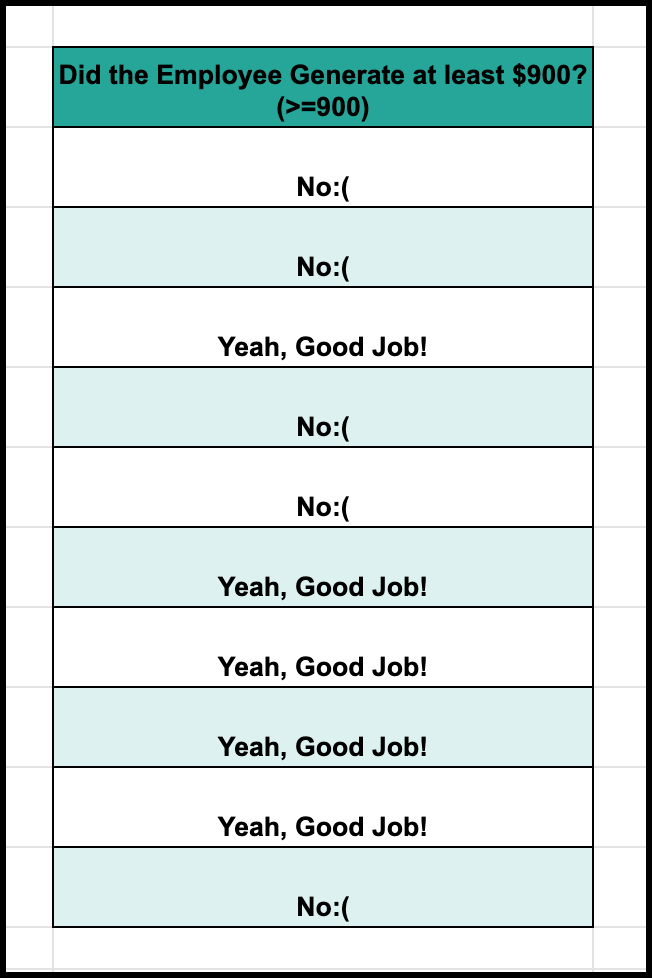The final step, as always, is to autofill your data.

You can apply this function to your entire dataset, and as you can see, you will now have one of two results appear dependent on your data. Always feel free to check your work and make sure that the function was correctly input.

## Deep(er) Dive

Now let's walkthrough a more complex example. The IF function is more versatile than a simple if-then-else, series.

### Compounding IF-Functions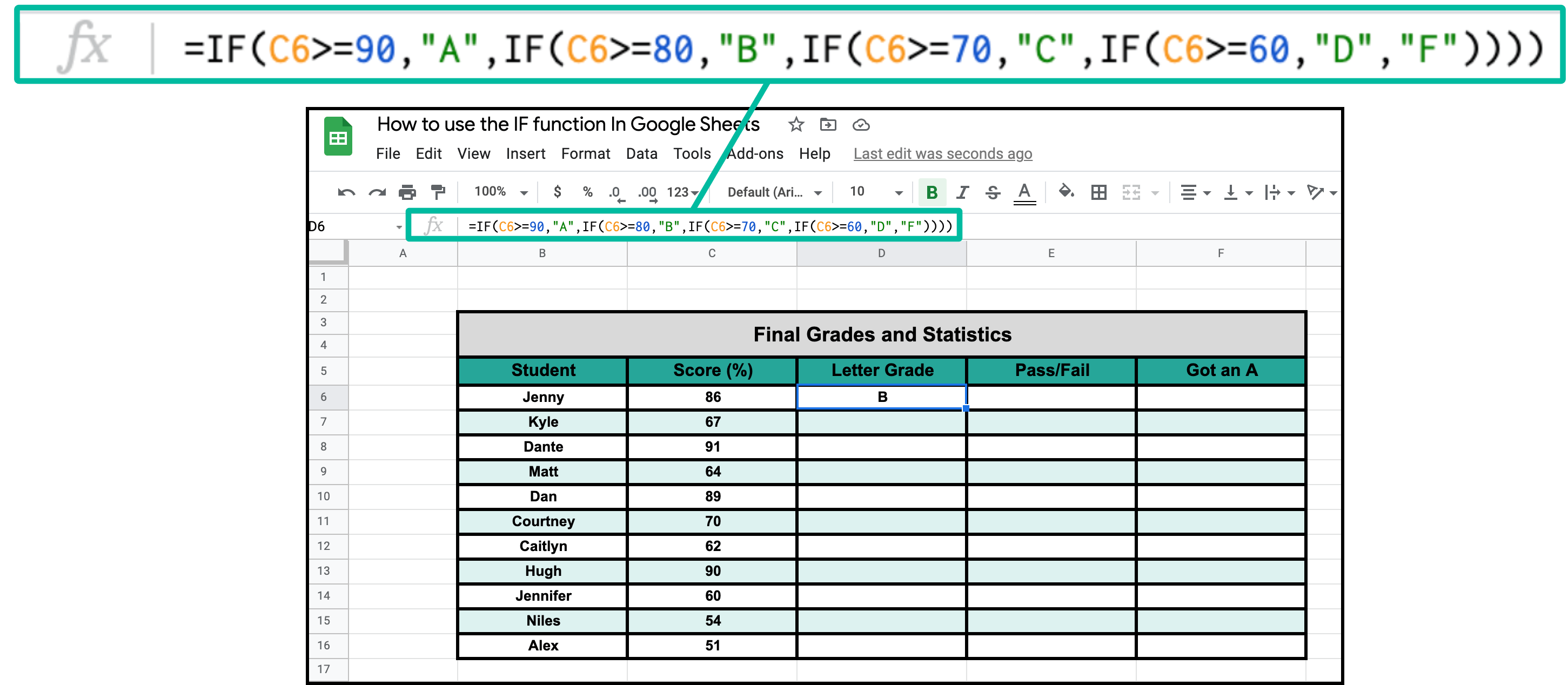Lets say you need Google Sheets to return more than two options in a given IF function. You can achieve this with what I call compounding IF-functions.

With this example, I am trying to return letter grades based on a students final percentage in my fictional class. As such, I will need a lot of criteria and limits to state. The cool thing about this tough, is that you can do it all within one function.

=IF(C6>=90,"A",IF(C6>=80,"B",IF(C6>=70,"C",IF(C6>=60,"D","F"))))

Instead of issuing a second option within my first IF statement, I have a new IF function within that one as the second option, and within that another, and on and on until I have covered all of my criteria.

### Give Your Sheets the Ability to "Rationalize"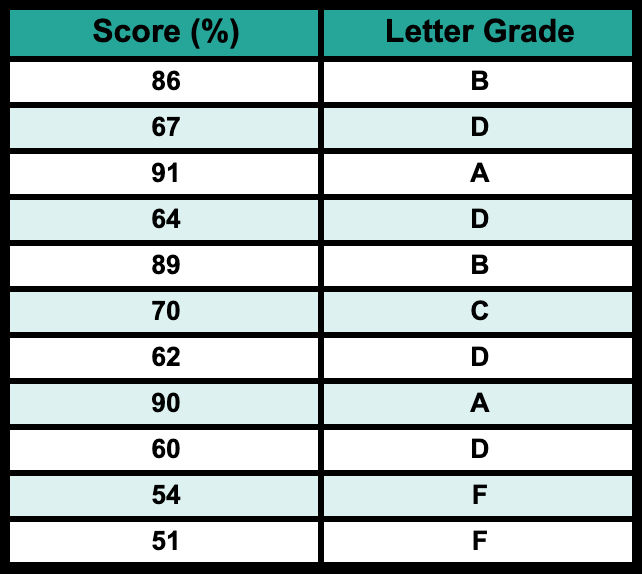In many ways, the IF function gives google sheets the ability to "rationalize". Within this example, the software will "think" in this order:

1. Is the number greater than or equal to 90? If yes, post "A" and end the scan, otherwise assume it's below 90 and go to step 2.
2. Is the number greater than or equal to 80? If yes, post "B" and end the scan, otherwise assume it's less than 80 and go to step 3.
3. Is the number greater than or equal to 70? If yes, post "C" and end the scan, otherwise assume it's less than 70 and go to step 4.
4. Is the number greater than or equal to 60? If yes, post "D" and end the scan, otherwise assume it's less than 60 and post the lowest option, "F".

Obviously this is very basic software, but I find this sort of thing astounding and incredibly interesting; you have so much versatility within Google Sheets that you can make google sheets process information and "think" if you really want it to.

Also, I would recommend using the IFS function for any compounded conditional functions like this; its just easier to visualize and execute, in my opinion.